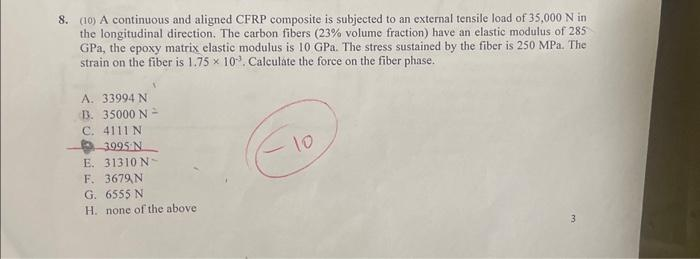# (Solved): 8. (10) A continuous and aligned CFRP composite is subjected to an external tensile load of 35,000 ...8. (10) A continuous and aligned CFRP composite is subjected to an external tensile load of in the longitudinal direction. The carbon fibers ( volume fraction) have an elastic modulus of 285 , the epoxy matrix elastic modulus is . The stress sustained by the fiber is . The strain on the fiber is . Calculate the force on the fiber phase. A. B. C. (s) E. F. G. H. none of the above 3

We have an Answer from Expert